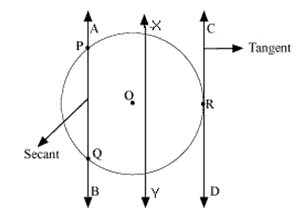# NCERT Solutions for Class 10 Maths Chapter 10 Circles Ex 10.1

NCERT Solutions for Class 10 Maths Chapter 10 Circles Ex 10.1 are part of NCERT Solutions for Class 10 Maths. Here are we have given Chapter 10 Circles Class 10 NCERT Solutions Ex 10.1.

Class 10th Math’s important questions in chapter Area Related to Circles for free download in PDF format. The most important questions for annual examination from chapter Introduction to Area Related to Circles are given here for download. Here you will find all the answers to the NCERT textbook questions of Chapter 10 Circles.

 Board CBSE Textbook NCERT Class Class 10 Subject Maths Chapter Chapter 10 Chapter Name Circles Exercise Ex 10.1 Number of Questions Solved 4 Category NCERT Solutions

## NCERT Solutions for Class 10 Maths Chapter 10 Circles Ex 10.1

Page No: 209

Question 1. How many tangents can a circle have?

Solution:
A circle can have infinite tangents.

Question 2. Fill in the blanks :
(i) A tangent to a circle intersects it in …………… point(s).
(ii) A line intersecting a circle in two points is called a ………….
(iii) A circle can have …………… parallel tangents at the most.
(iv) The common point of a tangent to a circle and the circle is called …………

Solution:
(i) one
(ii) secant
(iii) two
(iv) point of contact

Question 3.
A tangent PQ at a point P of a circle of radius 5 cm meets a line through the centre O at a point Q so that OQ = 12 cm. Length PQ is :
(A) 12 cm (B) 13 cm (C) 8.5 cm (D) √119 cm

Solution:PQ = √119 cm
Hence, (D) is the correct answer.

Concept Insight: To answer such type of problems, remember to use the result that the radius is perpendicular to the tangent at the point of contact and then make use of pythagoras theorem in the right traingle.

Question 4.
Draw a circle and two lines parallel to a given line such that one is a tangent and the other, a secant to the circle.

Solution:AB and CD are two lines parallel to the line XY. Line AB is intersecting the circle at exactly two points, P and Q. So, line AB is secant of this circle. The line CD is intersecting the circle at exactly one point, R. So, line CD is tangent to circle.

Concept Insight: To draw the two lines, remember that a tangent touches the circle at one point only and a secant intersects the circle at two distinct points.

We hope the NCERT Solutions for Class 10 Maths Chapter 10 Circles Ex 10.1 help you. If you have any query regarding NCERT Solutions for Class 10 Maths Chapter 10 Circles Ex 10.1, drop a comment below and we will get back to you at the earliest.